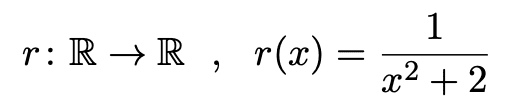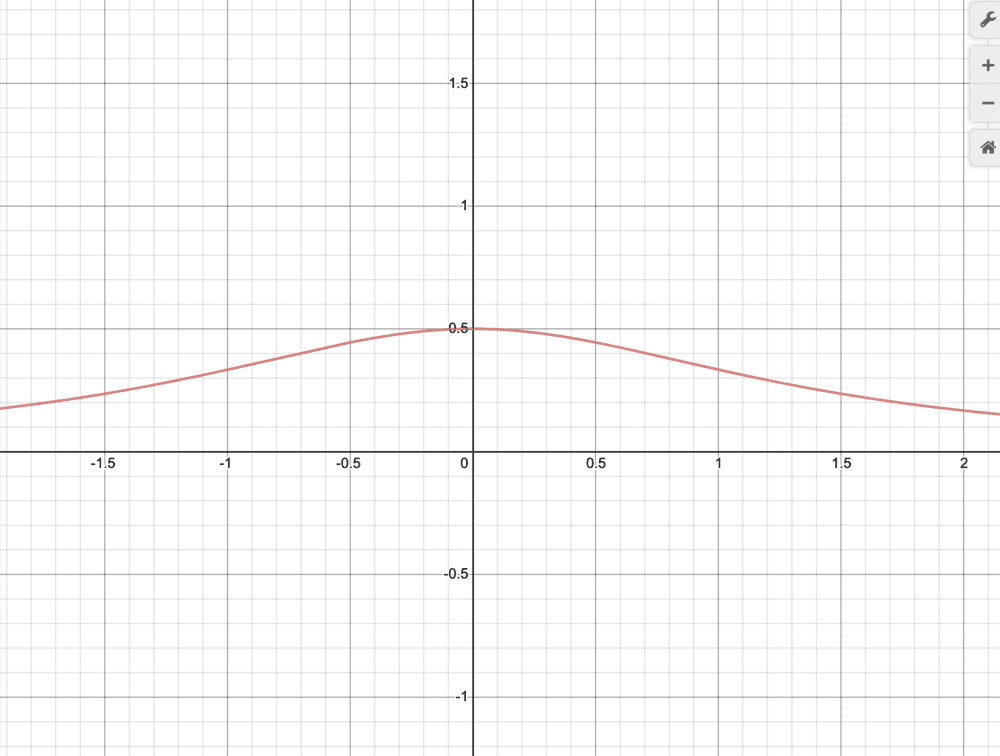# Find the images of the following function

angela107
Homework Statement:
I found the image of the following function (a graph). The question also asks me to explain my answer briefly. I'm not sure how to go about to answering.
Relevant Equations:
n/aMentor
Homework Statement:: I found the image of the following function (a graph). The question also asks me to explain my answer briefly. I'm not sure how to go about to answering.
Relevant Equations:: n/a

View attachment 270198

View attachment 270199
So just start talking about how the equation produces the graph. What is the value of the function with x=0? Why is that the maximum that the function can produce? Why is the graph symmetric about the x=0 axis? With an x in the denominator, why is the function not equal to infinity somewhere?

angela107
So just start talking about how the equation produces the graph. What is the value of the function with x=0? Why is that the maximum that the function can produce? Why is the graph symmetric about the x=0 axis? With an x in the denominator, why is the function not equal to infinity somewhere?
Thank you!

Mentor
Thank you!
You're welcome. And your thoughts are...